CHEMISTRY As LEVEL(FORM FIVE) – GENERAL CHEMISTRY (2)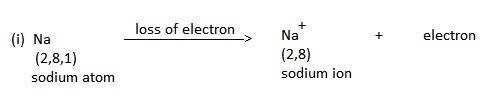Written by

ecolebooks.com

ELECTROVALENCY
The number of electrons lost or gained by an atom of any element is termed as electrovalency. The element which gives up the electron(s) to form positive charge or ions are said to have positive valency. While the element(s) which accepts electrons to form negative ions are said to exhibit negatively valency.

Note:- Variable electrovalency of iron exist as Fe2+ and Fe3+ in ferrous sulphate and ferric sulphate .when a compound is formed by the transfer of electrons, the element that loses electron(s) is said to be oxidized and the element that gains electrons is said to be reduced.
Oxidation is a process which involves loss of electrons where as reduction is the process which involves gaining of electrons.
PROPERTIES OF IONIC/ELECTROVALENT BOND
An ionic/Electrovalent bond has the following properties:-
(i) An ionic bond is formed due to the coulombic attraction between the positively and negatively charged ions.
(ii) An ionic bond is non-directional, the strength of interaction between two ions depend upon distance but not the directional.
(iii) An ionic bond gets broken when the substance is dissolved in polar solvent such as water or when the substance is melted.

Typical examples of ionic bond:-
(a) Na + Cl → NaCl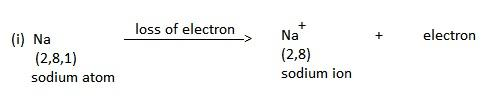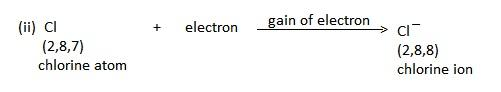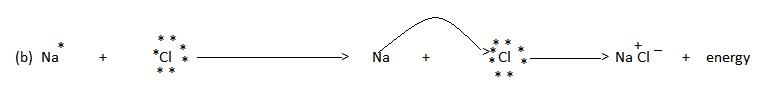FACTORS INFLUENCING THE FORMATION OF IONIC BOND
The main steps involved in the formation of an electrovalent/ ionic bond are:-
(i) Removal of electrons from one atom, in this stage energy equal to the ionization energy is absorbed.
(ii) Gaining of electrons by the other atoms .In this step energy equal to the electrons affinity is released.

(iii) Combination of cation and anions. These ions are held together by coulombic force of attraction. In this step, energy equal to the lattice energy is released.

(II) COVALENT BOND
A covalent bond is formed between two atoms (similar and dissimilar) by a mutual sharing of electrons .the shared pairs of electrons are counted towards the stability of both the participating atoms.

DEFINITION: – A covalent bond is defined as the force of attraction arising due to mutual sharing of electrons between the two atoms.
When the two atoms combine by mutual sharing of electrons, then each of the atoms acquire stable configuration of the nearest noble gas.

COVALENCY
Is the number of electrons which an atom contributes towards mutual sharing during the formation of a chemical bond.
Example of the Covalency: – Covalency of hydrogen (H2)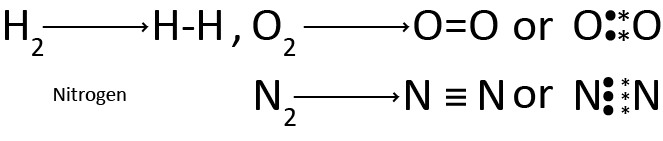CHARACTERISTICS OF COVALENT BOND
(i) Mode of formation, covalent bond are formed due to mutual sharing of one or more pairs of electrons.
(ii) Directional character , covalent bonds are directional in nature this is because the shared electrons remains localized in a definite space between the nuclei of the two atoms.This gives a directional character to the covalent bond.
SINGLE COVALENT BOND
A covalent bond formed by mutual sharing of one pair of electrons. A single covalent bond is represented by a small line (-) between the two atoms.
E.g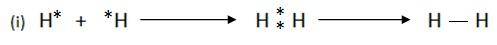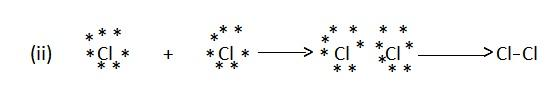MULTIPLE COVALENT BONDS
The covalent bonds developed due to mutual sharing of more than one pairs of electrons are termed as multiple covalent bond.
The multiple covalent bonds are:-
(i) Double covalent bond
(ii) Triple covalent bond
Double covalent bond is the bond formed between two atoms due to the sharing of two electrons pairs. Its simply called Double bond

E.g. O2 → O = O
CO → C = O etc.

Triple covalent bond is the bond formed due to the sharing of three electron pairs
E.g N2 → N ≡ N,
Acetylene H – C ≡ C – H.

(I) FORMATION OF MOLECULES HAVING DOUBLE BOND.
(i) Formation of oxygen (O2) molecule:-
Each oxygen atom has six electrons in its outer most shell. Thus it requires two more electrons to achieve the nearest noble gas configuration.
E.g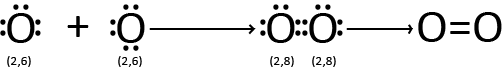(ii) Formation of carbon dioxide(CO2) gas
The electronic configuration of carbon and oxygen are :-

C 1s2 2s22p2 2,4
O 1s2 2s22p4 2,6

Thus each carbon atom requires four ,and each oxygen atom requires two more electrons to acquire noble gas configuration.This is achieved as follows
e.g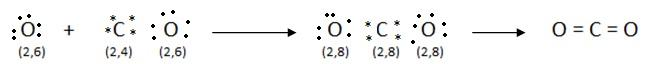(iii) Formation of molecules having triple bond :-
(a) Formation of nitrogen (N2) molecule.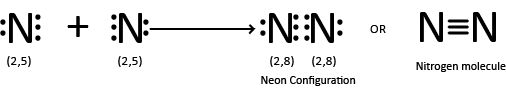COMPARISON BETWEEN SINGLE,DOUBLE AND TRIPLE COVALENT BONDS
Triple bond length < Double bond length < Single bond length.
Since a shorter bond means greater bond strength hence the energy required to separate the bonded atoms( called bond energy) follows the order
Triple bond > Double bond > Single bond

FACTORS FAVOURING THE FORMATION OF COVALENT BOND
The following are the factors that favour the formation of a covalent bond:-
(i) High ionization enthalpy (energy).
The element having higher ionisation enthalpy (energy) cannot lose electrons easily.

(ii) Nearly equal electron gain enthalpy or electron Affinity.
The atoms of the two elements which have equal or nearly equal electron gain enthalpies or electrons affinities tend to complete their outer shells by mutual sharing of electrons.

(iii) Nearly equal electronegativity.
Equal or nearly equal electronegativity of the two combining elements does not permit the transfer of electron(s) from one atom to another.

(iv) High nuclear charge and small atomic size.
High nuclear charge, and smaller atomic size of the combining elements favour covalent bond formation, because the transfer of electrons in such case will not be possible.
COORDINATE COVALENT BOND
Coordinate bond is formed when the shared electron pair is provided by one of the combining atoms.The atom which provides the electron pair is termed as the donor atom ,while the other atoms which accept is termed as the acceptor atom
The bond formed when one sided sharing of electrons take place is called a coordinate or Dative bond. A coordinate bond is presented by an arrow ( → ) pointing towards the acceptor atom.

(i) Formation of coordinate bond during the formation of a molecule or molecular ion:-
(a) Formation of Ammonium (NH4+) ion.
During the formation of ammonium ion ,nitrogen is the donor atom while H+ is the acceptor ion.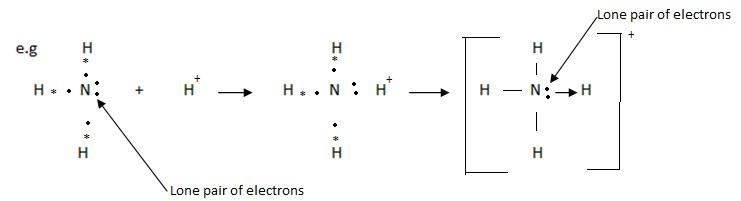(b) Formation of coordinate bond between two molecules:-
Two or more stable molecules combine to form a molecular complex,is such a complex molecule, the constituent molecules are held together by coordinate bond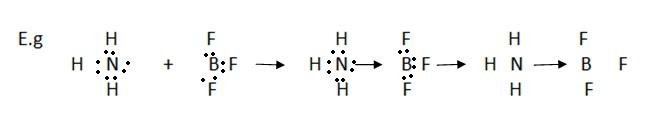The product above is ammonia-borontrifluoride complex
POLARITY IN COVALENT BONDS :-
Depend upon chemical nature of the combining elements, the following two types of covalent bond are formed

(i) Non-polar covalent bond
(ii) Polar covalent bond
(i) NON-POLAR COVALENT BOND
When a covalent bond is formed between two atoms of the same element, the electrons are shared equally between the two atoms .The resulting molecule will be electrically symmetrical.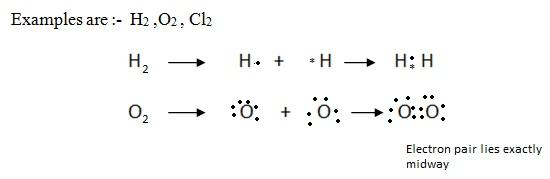(ii) POLAR COVALENT BOND
When a covalent bond is formed between two atoms of different elements, the bonding pair of electrons does not lie exactly midway between the two atoms. It lies more towards the atom which has more affinity for electrons.
The atom with higher affinity for electrons thus develops a slight negative charge and the atom with lesser affinity for electrons a slight positive charge, such molecules are called polar molecules.The covalent bond between two unlike atoms which differ in the affinities for electrons is said to be a polar covalent bond.
Example of polar covalent bond are hydrogen chloride (H Cl) , water (H2O)
CAUSES OF POLARITY IN BONDS
The cause of polarity in bond is due to the electronegativity difference.
Example of the electronegativity difference between hydrogen and halogen atoms is as follows:-
halogen atoms: (F,Cl,Br,I)
electronegativity differences:-

H – F > H – Cl > H – Br > H – I
(4- 2.1) (3.0- 2.1) (2.8- 2.1) (2.5- 2.1)
1.9 0.9 0.7 0.4

VALENCE SHELL ELECTRON PAIR REPULSION (VSEPR) THEORY.
The VSEPR theory was proposed by R.J Gillespie and R.S Nyholmn in 1957. This theory was developed to predict the shapes of the molecule in which the atom are bonded together with single bond only. This theory is based on the repulsions between the electron pairs in the valence shell of the atoms in the molecule. The main postulate of the vsepr theory are:-
(i) The geometry of a molecule is determined by the total number of electron pairs (bonding and non-bonding) around the central atom of the molecule.
The shape of the molecule depends upon the orientation of these electron pairs in the space around the central atom.
(ii) The electron pairs (shared or lone pairs) around the central atom in a molecule tend to stay as far away from each other as possible so as to minimize the repulsion forces
between them.
(iii) The strength of repulsion between different electron pairs follows the order.

Lone pair-lone pair > lone pair-shared pair > shared pair-shared pair
The shared pair of electrons are also called bond pair of electrons.The presence of lone pair(s) of electrons on the central atom causes some distortions in the expected regular shape of the molecules.

PREDICTING THE SHAPE OF MOLECULES ON THE BASIS OF VESPR THEORY
(i) Molecules with two bond pairs
In a molecule having two bonds pairs of electrons around its central atom, the bond pairs are located in the opposite side (at an angle of 180º) of the central atom so that the repulsion between them is minimum.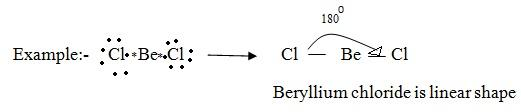(ii) Molecules with three bond pair
In a molecule having three bond pairs of electrons around its central atom, the electron pair form an equilateral triangular arrangement around central atom. Thus the three bond pairs at 120º with respect of each other. Thus the molecule having three bond pairs around its central atom have trigonal planar (triangular planar shape).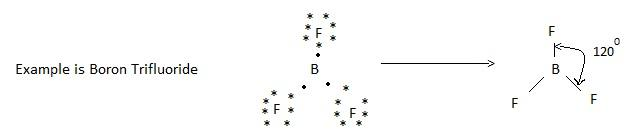(iii) Molecules with four bond pairs
In a molecule having four bond pairs of electrons the four bond pairs are arranged tetrahedral around the central atom. Thus the four band pairs are at an angle pf 129º 28’ with respect to each other. Therefore the molecule having four bond pairs around its central atom has a tetrahedral shape.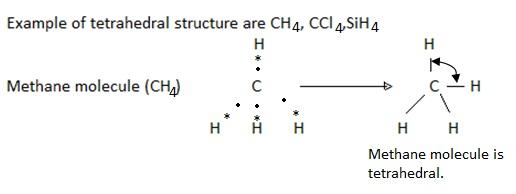(iv) Molecules with five bond pairs
Five bond pair orient themselves around the central atom in a trigonal bipyramidal way. Three bond pairs are in a plane called equatorial plane, and oriented at an angle of 120º with respect to each other.
Thus a molecule having five bond pairs around its central atom has a triangular bipyramidal shape.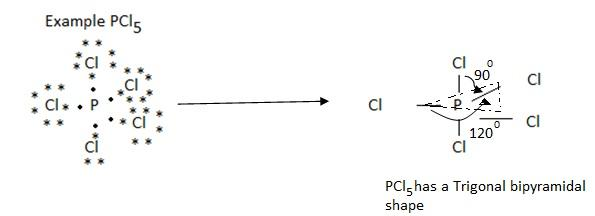Therefore the molecule of the type shape AB5 are trigonal bipyramidal in shape e.g PCl5, PF5, SbCl5.
(v) Molecules with six bond pairs
Six bond pairs in a molecule are distributed octahedral around the central atom. Thus a molecule having six bond pairs around its central atom has an octahedral shape. Thus the molecules of the type AB6 are octahedral. Thus molecules SF6 has an octahedral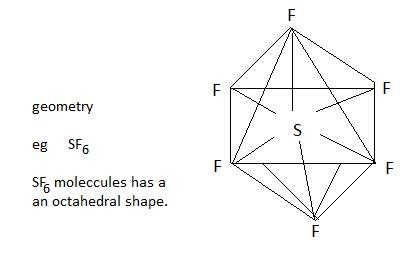SHAPES OF THE MOLECULES HAVING BOND PAIRS AND LONE PAIR ELECTRONS.
The pair of electrons in the valence shell of an atom which is not involved in bonding is called lone pair of electrons.
For example the nitrogen atom in ammonia molecules (NH3) has one lone pair of electrons, the oxygen atom in water molecule (H2O) has two lone pairs of electrons.
(i) Molecules having three bond pairs and one lone pair.
A molecule having three bond pairs and one lone pair of electrons, thus has in all four pairs of electrons around its central atom.
Therefore these four pairs of electrons are distributed tetrahedral around the central atom.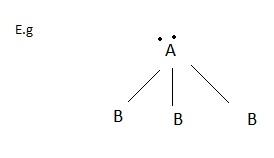(ii) A molecule of AB3 type has a triangular pyramidal shape
Typical molecules of this type are NH3, NF3,PCl3 and H3O+.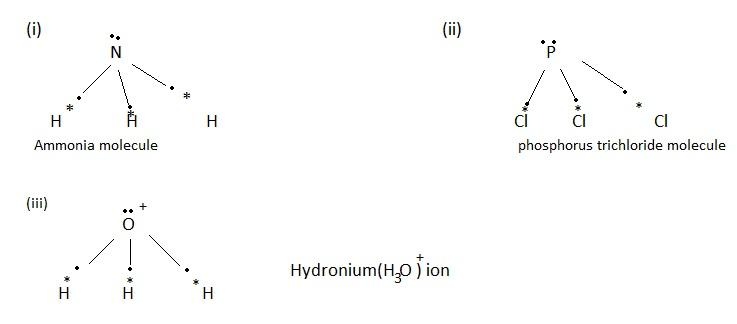(iii) Molecules with two bond pairs and two lone pairs
The four electron pairs (two bond pair + two lone pair ) are distributed tetrahedral around the central atom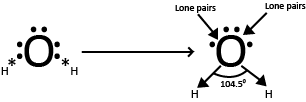The two lone pairs are the central atom repel the bond pairs slightly inward due to greater lone pair-bond pair repulsion. As a result the bond angle in such a molecule is less than the tetrahedral vale of 109º 28’
The presence of only two bond pairs is the molecules gives a bent shape (inverted V- shaped) to the molecule. Example include H2O, H2S, F2O and SCl2.
(iv) Molecules with four band pair and two lone pairs
The four bond pair are distributed in a square planar distribution. The two lone pairs are in a direction at right angles to this plane.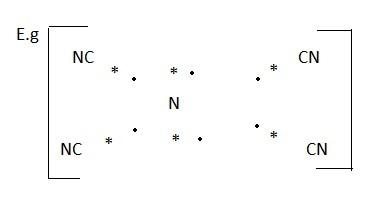EXERCISE:-
(i) predict the shapes of the following molecules following the VSEPR theory.

(a)Ammonia (NH3) molecules
(b)beryllium chloride(BeCl3)
(c)water (H2O) molecule
(d)silicon tetrachloride (SiCl4)
HYBRIDIZATION
Definition:- Is the process of mixing of the atomic orbitals to form new hybrid orbital.
Note:- All hybrid orbitals of a particular kind have equal energy, identical shape and are symmetrically oriented in space.
CHARACTERISTICS OF HYBRID ORBITALS
(i) The number of hybrid orbitals formed is equal to the number of the atomic orbitals participating in hybridization.
(ii) All hybrid orbitals are equivalent in shape and energy but different from the participating atomic orbitals.
(iii) A hybrid orbital which takes part in the bond formation must contain only one electron in it.
(iv) A hybrid orbital, like atomic orbital cannot have more than two electrons. The two electrons should have heir spins paired.
(v) Due to the electronic repulsions between the hybrid orbitals they tend to remain at the maximum distance from each other.
TYPES OF HYBRIDIZATION
The types of hybridization shown by an atom depends upon the requirement of the reaction.
(a) SP3(ess-pee three) hybridization (or tetrahedral hybridization)
(b) sp2(ess-pee two) hybridization (or trigonal hybridization)
(c) sp (ess-pee ) hybridization (or linear hybridization)
(d) s,p & d) hybridization
(a) SP3 HYBRIDIZATION
→ S + 3-P orbitals under mixing to provide new hybrid orbitals.
e.g S + (Px+ Py+ P2) → SP3
one s- orbital three p- orbital four hybrid orbital
Each of these hybrid orbitals has 25% S-character and 75% P-character. These four sp3 hybridized orbitals are directed along the four corner of a tetrahedron and are inclined to each other at an angle 109º 28’
Examples of sp3 hybridization is that of methane (CH4), the process of hybridization involves the following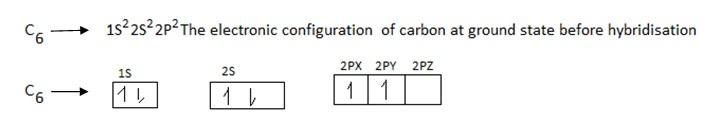During exited state of carbon one electron is promoted from 2S to 2P orbitals .The process of promotion of an electron from 2s to 2p orbital of the carbon atom and subsequent hybridization is illustrated below.
During hybridization/ During excited state ,S is promoted to P orbitals as shown below:Shape of S is spherical and shape of p is damp bell, then S hybridized with p shape.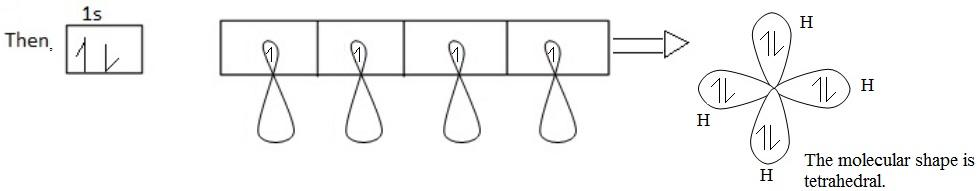Hybrid between S + P(b) SP2 HYBRIDIZATION (trigonal hybridization)
Example of SP2 hybrid orbital are the formation of ethane molecule(C2H4)
Note:- The three SP2 hybrid orbitals are oriented in a plane along the three corners of an equilateral triangle .i.e. they are inclined to each other at an angle of 120º the third p – orbital (say P2) remains unchanged each hybrid orbital has 33.3% s – character and 66.7% p – character
SP2 hybridization of Ethene (C2H4)/Ethylene
(i) Structure of ethane.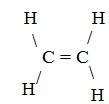(ii) Electronic configuration of carbon.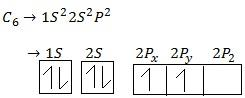(iii) During excitation hybridization takes place. Then;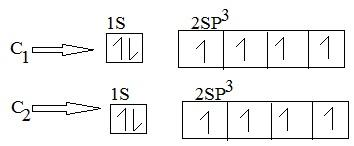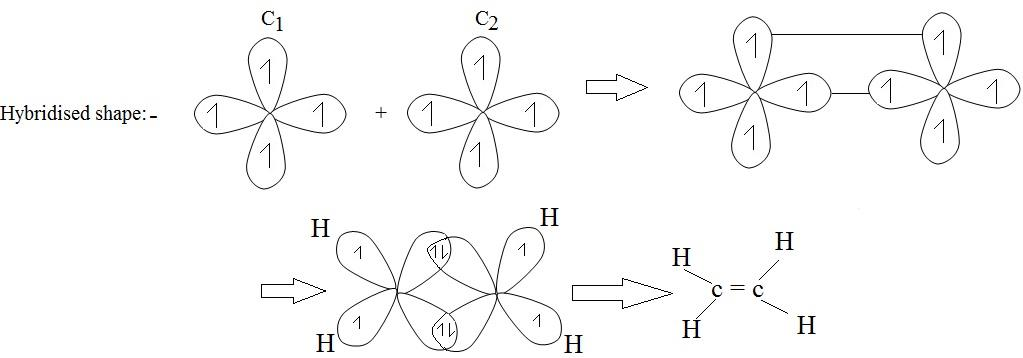(c) SP hybridization(or linear hybridization)
Example of SP hybridization is the formation of ethyne(acetylene) molecules(c2h2)
(i) Structure of ethyne (acetylene)molecule
E.g (i)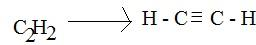(ii) Electronic configuration of carbon from ground state.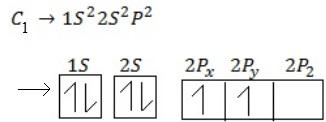(iii) During excited state , S is promoted to P – orbital to form hybrid orbital.

(a)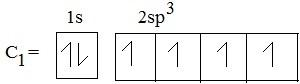Hybrid shape for carbon 1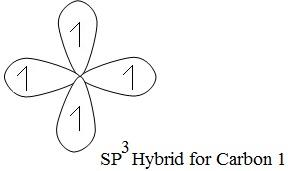(b)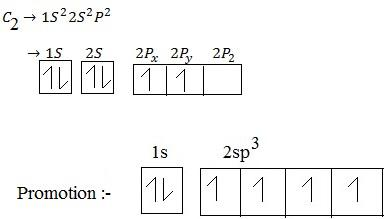Hybrid shape for carbon 2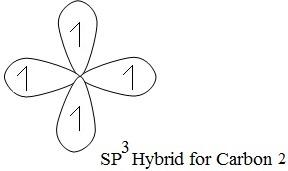Then C1 combine with C2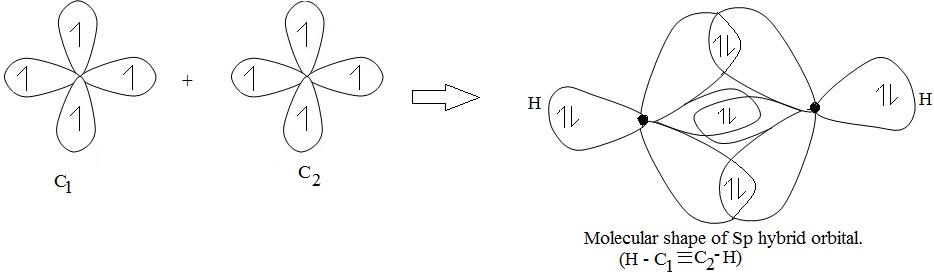HYDROGEN BONDING
If a hydrogen atom is bonded to a highly electronegative element such as fluorine , oxygen, nitrogen, then the shared pair of electrons lies more towards the electronegative element. This leads to a polarity in the bond in such a way that w slight positive charge get developed on H- atom.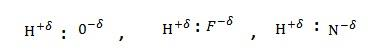The bond between the hydrogen atom of one molecule and more electronegative atom of the same or another molecule is called hydrogen bond
CONDITION NECESSARY FOR THE FORMATION OF HYDROGEN BOND
(i) Only the molecule in which hydrogen atom is linked to an atom of highly electronegative element are capable of forming hydrogen bond.
(ii) The atom of highly electronegative element should be small.
SOME TYPICAL COMPOUND SHOWING HYDROGEN BONDING
(i) Hydrogen fluoride( HF)
Structure of hydrogen fluoride:-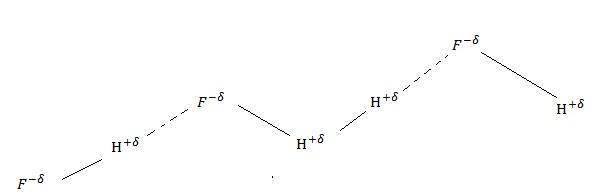(ii) Water (H2O).
Water is a typical polar compound which exhibit hydrogen bonding as shown below:-
Structure of water molecule.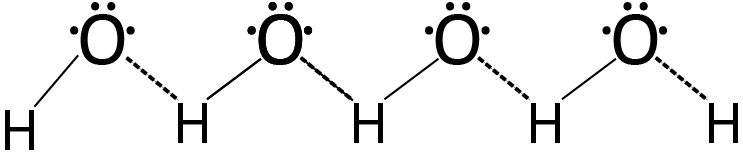Hydrogen bonding that water exist in the associated form
TYPE S OF HYDROGEN BONDING
There are two types of hydrogen bonding
(i) Intermolecular hydrogen bonding
(ii) Intramolecular hydrogen bonding
(i) INTERMOLECULAR HYDROGEN BONDING.
When the hydrogen bonding is between the h-atom of one molecule and an atom of the electronegative element of another molecule.
For example , hydrogen bonding in water, ammonia etc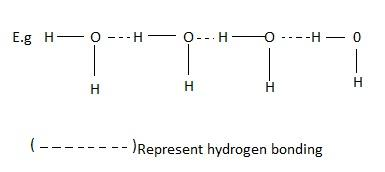(ii) THE INTRAMOLECULAR HYDROGEN BONDING
Is between the hydrogen of one functional group and the electronegative atom of the adjacent functional group in the same molecule. For example the molecule of o-nitrophenol show intramolecular hydrogen bonding. The p-nitrophenol shows intermolecular hydrogen bonding.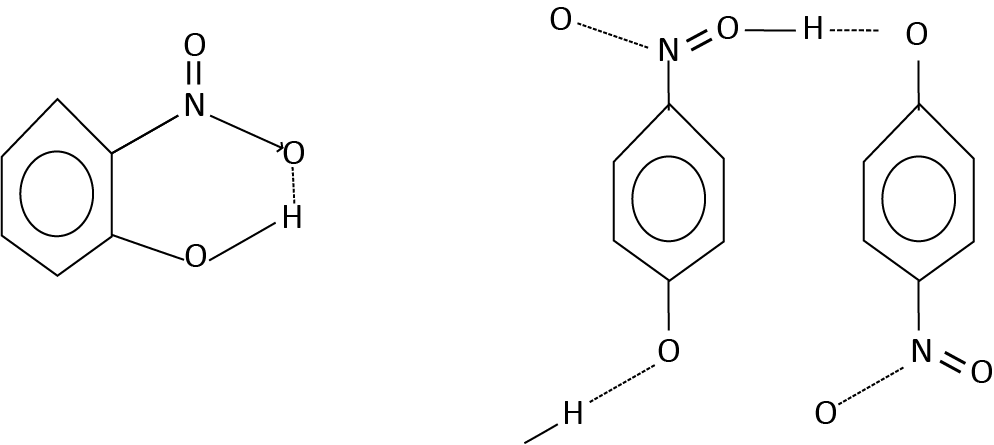EFFECT OF HYDROGEN BONDING
Hydrogen bonding affects the physical properties of the compound appreciable .
Some effect of hydrogen bonding are described below:-
(i) Molecular association.
Formation of aggregates containing two or more molecules due to weak electrostatic interactions such as hydrogen bonding for example ,water molecule undergoes molecular association due to hydrogen bonding.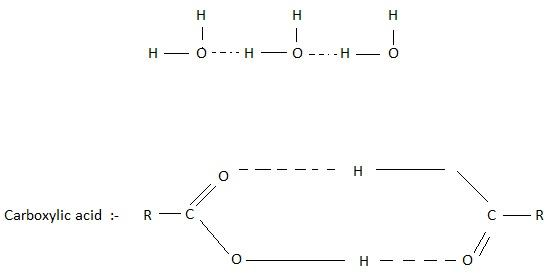(ii) Increase in the melting and the boiling points.
The interaction which affected the melting and boiling points of NH3, H2O and HF is identified to be hydrogen bonding.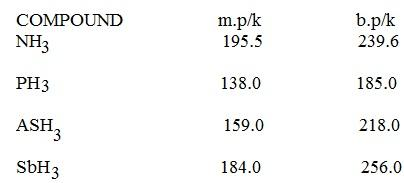(iii) Influence on the physical state.
Hydrogen bonding affects the physical state of a substance also for example H2O is liquid while H2S is a gas under room temperature condition

(iv) Solubility of covalent compound in water
Covalent compounds generally do not dissolve in water. However the covalent compound which can form hydrogen bonds with water readily dissolved in it. For example ethanol, Ammonia, Ammine lower aldehyde and Ketones dissolve in water due to their tendency to form hydrogen bond with water.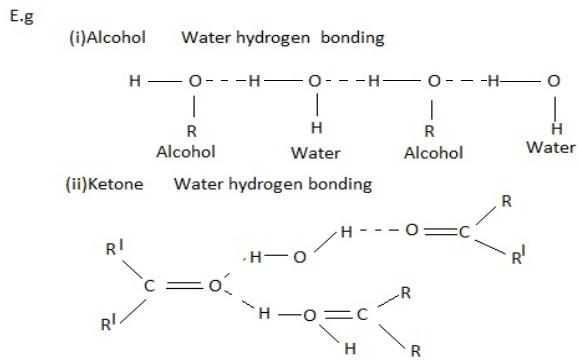REVIEW QUESTIONS
Question 31
(a) Using the electronic configuration and periodic table, give the name of the element and the number of valence electrons.
(i) 1s2 2s2 2p4
(ii) 1s2 2s2 2p6 3s2 3p3
(b) Use Hund’s rule and other information to write out electronic configuration and orbital diagram of;-
(i) Cobalt
(ii) Nickel
(iii) Zinc
Solution
(a) (i) Oxygen: It has 6 valence electrons in the 2s and 2p sub levels.
(ii) Phosphorus: It has 5 valence electrons (in the 3s and 3p sub level)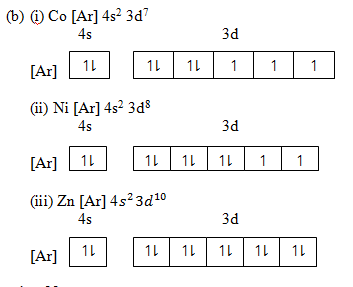Question 32
(a) Provide the number of orbital in each of the following shells;-
(i) 1s
(ii) 2p
(iii) 3d
(iv) 4f
(b) Howe many sub – shells are there in each of the following sub – shells? K, N and L
(c) Explain if it is possible for an electron to have the following set of quantum numbers.
n = 3, â„“ = 3; m; m = 3 and s = +1/2
(d) An electron is in a 4f orbital. What possible value for the quantum number can it have?
(e) What sub shell is found in the shell with n value = 4
(f) Write the possible value of the 4 quantum number for the outermost 2 electrons of Calcium.
(g) Write all the four quantum number for an electron added to Cl atom in forming Cl
(h) Write all the four quantum numbers for the outmost 2 electrons in Na
Question 33
(a) Write the total number of electrons possible for an atom whose n = 3. Assume that all the orbitals are full of electrons.
(b) Write electronic configuration of the following elements/ions
(i) O
(ii) K
(iii) Ni at number 28
(iv) Cu+ (at number of Cu = 29)
(v) Mo at number 42
(vi) Cl (at number of Cl = 17
(c) What is the number of unpaired electrons present in the ground state of;-
(i) Fe+3 (At NO. of Fe = 26)
(ii) Cr3+ (At No. of Cr = 24)
(iii) Ni2+ (At No. of Ni = 28
(d) Why is the electronic configuration 1s2 2s2 2p2x 2p0y 2p0z not correct for the ground state of nitrogen?
(e) Mention the disobeyed law from the following arrangements;-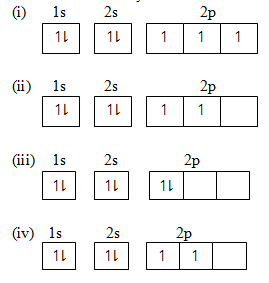Question 1
(a) State two major postulates and five short coming of Bohr’s atomic models.
(b) An electromagnetic radiation was emitted in the Balmer’s series as a result of electron.
Transitions between n= 2 and n= 5 calculate the;
(i) Energy of the radiation in kJmol-1
(ii) Frequency of the radiation in hertz
(iii) Wavelength of the radiation in metres.
(c) Calculate the wavelength of a line in the Balmer Series that is associated with energy transition
E4 E2 (E4 = -1.362 x 10-19J; E2 = -5.448 x 10-19J; h = 6.63 x 10-34J; C = 3 x 10-8 m/s)

Question 9
(a) The atomic spectrum in the visible is given by the following relationship: = RH
(i) What do symbols λ, RH, n1 and n2 represent? Show their SI units
(ii) Calculate the frequency of the third line of the visible spectrum i.e. a transit form n = 5 to n = 2 (RH = 1.097 X 107 M-1)
(b) By means of series of horizontal lines, draw an energy level diagram for visible atomic spectrum of hydrogen and indicate:
(i) The value of n for each line
(ii) By means of an arrow, show the transition which corresponds to the fourth line of emission spectrum in visible region.
(iii) By means of an arrow, indicate the transition which corresponds to the fourth ionization energy of hydrogen atom.
(c) (i) What do you understand by the term convergence limit and what is its significance?
(ii) Why are the discrete lines observed and not a continuous spectrum in Hydrogen spectrum?
Question 25
(a) Define the term electron configuration.
(b) Write down the electron configuration of these elements in their ground;
(i) Hydrogen 1p.
(ii) Beryllium.
(iii) Neon 10p.
(iv) Aluminium 13p.
(v) Calcium 20p.
(vi) Manganese 25p.
(vii) Cobalt 27p.
(viii) Zinc 30p.
(ix) Krypton 36p.
(x) Silicon 14p.

Question 18
Write electronic configuration diagram of the following;-
(a) S-2 (b) Na+ (c) Cr+3 (d) Cr+6 (e) Zn+2
Question 15
Write the electronic configuration of the following;
25V = [Ar] 4s2 3d3
16S = [Ar] 3s2 p4
9F = [He] 2s2 p5
34Cs = [Ar] 4s2 d10 4p4
16In = [Kr] 5s2 4d8
26Fe = [Ar] 4s2 d6
56Ba = [Xe] 6s2
Question 16
Provide electronic configuration of the following;
75Pt
80Br
68I
48Ga
92Cf
58Te
102Ra
17Cl
15P
30Zn
Question10
(a) All radiations are associated with the wave nature and differ from one another terms of their wavelength. Frequency, velocity and energy. Give the relationship between;
(i) Frequency and wavelength.
(ii) Energy and frequency.
(iii) Energy and wavelength.
(b) Ozone (O) protects the earth’s inhabitants from the harmful effects whose wavelength is 2950A. Calculate
(i) Frequency.
(ii) Energy.
for this UV light
(c) If the wavelength of the first line of the Balmer series in a hydrogen spectrum is 6563, calculate the wavelength of the first line of Lyman series in the same spectrum. The uncertainty of the momentum of particle is 3.3 x 10-16gms-1. Find the accuracy in which its position can be determined.
Question 11
a) (i) A small object of mass 10g is thrown with velocity of 200 m/s given h = 6.626 x 10-34J.S. Calculate its wavelength.
(ii) Kinetic energy of a sub – atomic particles is 5.65 x 10-23J. Calculate the frequency of the particle wave (h = 6.626 x 10-34)
(iii) Calculate the momentum of a particle which has a de-Broglie wavelength of 0.1nm (h = 6.6 x 10-34 JS).
(iv) Two particles A and B are in motion, if wavelength λ of A is 5 x 10-8m. Calculate the wavelength λ of B. if its momentum is half that of A.
b) State and describe briefly Heisenberg uncertainty principle.
c) (i) Describe the concept that as the particle size gets smaller determination of its momentum and position simultaneous becomes difficult
(ii) Use the mathematical expression of the uncertainty principle to prove the validity of part c (i) above
d) (i) Calculate the uncertainty in position of an electron if uncertainty in velocity is 5.7 x 105 m/s
(ii) The uncertainty in position and velocity of a particle are 1010m and 5.27 x 1024ms-1 respectively. Calculate the mass of the particle.

Question 12
(a) Outline the postulates and weaknesses of Bohr’s atomic model.
(b) Calculate the mass of a photon of sodium light having wavelength of 5894A and velocity 3 x 108m/s given h = 6.6 x 10-34kgm2s-1 and 3.7 x 10-36kg.
(c) What’s the wavelength of a particle mass 1 gram moving with a velocity of 200ms-1 given h = 6.626 x 10-34J. (Answer 3.31 x 10-29m)
(d) The mass of an oxygen molecule is 5.3 x 10-26kg. Calculate its de Broglie wavelength if it is moving at a speed of 500m/s (Planks constant (h) = 6.626 x 10-34Js (Answer 2.5 x 10-11m)

Question 13
(a) Briefly explain the meaning of these terms, with the help of a diagram
(i) Wavelength
(ii) Frequency
(iii) Velocity
(iv) Amplitude
(v) Wave number
(b) Yellow light from sodium lamp has a wavelength of 5800Ao. Calculate the frequency and wave number of the radiations. Determine the range of frequency of visible light. The wavelength of radiations lying in the visible regions are between (3800 – 7600A0).
Question 14
(a) (i) What do you understand by dual nature of matter?
(ii) How does de Broglie equation consider the dual nature of matter?
(b) (i) Calculate and compare the energies of two radiations, one with wavelength 800nm and the other with 400nm
(ii) What is the amount of radiant energy associated with atomic 6.662 x 10-34Js, velocity of light 3 x 108m/s
(c) If the wavelength of a beam of light is 2.8 x 10-7m. Calculate it’s;-
(i) Wavelength in cm.
(ii) Frequency.
(iii) Energy of one of its photons.
(d) (i) Referring to Bohr’s atomic model, what’s ionization energy?
(ii) Calculate ionization energy of hydrogen. Reydberg’s constant RH = 1.097 x 107m-1
(e) (i) Calculate the frequency of the 3rd line of visible spectrum.
(RH=1.097 x 107m, C=3 x 108m/s)

(ii) Given that the wavelength of the 1st line of Balmer series is 6563A. Calculate the wavelength of the 2nd line in the spectrum.